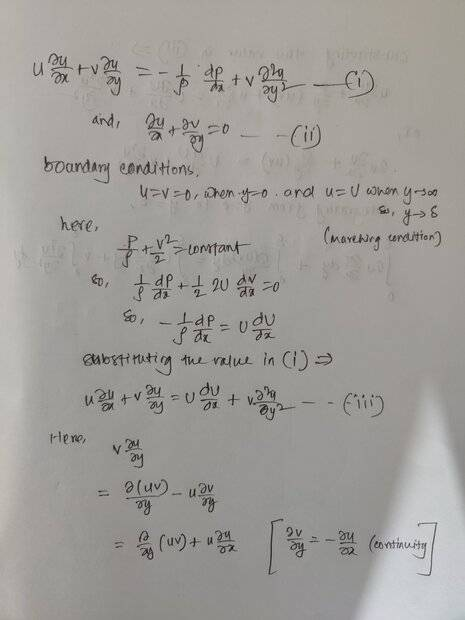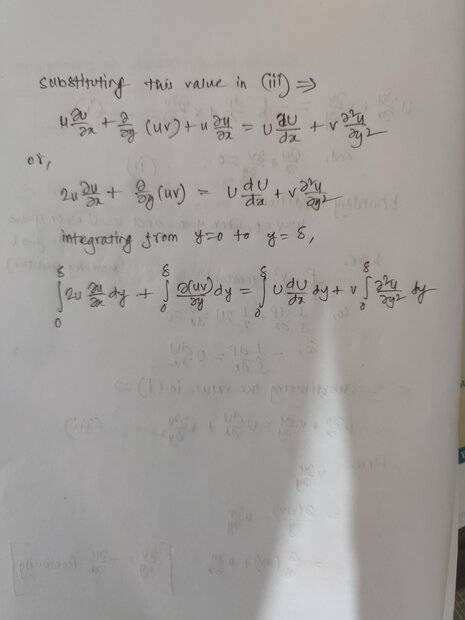# Fluid dynamics problem involving the Prandtl boundary layer equation for a two dimensional steady laminar flow

• Engineering
• sakif

#### sakif

Homework Statement
Prove the following statement
Relevant Equations
Prandtl boundary layer equation for a two dimensional steady laminar flow of incompressible fluid over a semi infinite plate
I have tried to approach in the following way#### Attachments

•berkeman
I see you try to use the Bernoulli equation. But that is never going to work since that equation is only valid for inviscid flow. And in an inviscid flow no boundary layer can exist.

Arjan82 said:
I see you try to use the Bernoulli equation. But that is never going to work since that equation is only valid for inviscid flow. And in an inviscid flow no boundary layer can exist.
Far from the boundary, in the free stream, it is valid to use the Bernoulli equation. That's what boundary layer theory is all about.